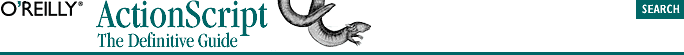home | O'Reilly's CD bookshelfs | FreeBSD | Linux | Cisco | Cisco Exam## 4.3. Numeric Literals

### 4.3.1. Integer Literals

We use a leading zero to indicate an octal number. For example, to represent the octal number 723 in ActionScript, we use:

`0723  // 467 in decimal (7*64 + 2*8 + 3*1)`

To indicate a hexadecimal (hex for short) literal integer, we put 0x (or 0X) in front of the number, such as:

```0x723  // 1827 in decimal (7*256 + 2*16 + 3*1)
0xFF   //  255 in decimal (15*16 + 15*1)```

#### Example 4-1. Trim Leading Zeros

```function trimZeros(theString) {
while (theString.charAt(0) == "0" || theString.charAt(0) == " ") {
theString = theString.substring(1, theString.length);
}
return theString;
}

testString = "00377";
trace(trimZeros(testString));  // Displays: 377```